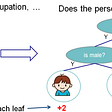# Types of Linear Regression

1. Simple Linear Regression

# 2. Multiple Linear Regression

• y = the predicted value of the dependent variable
• B0 = the y-intercept (value of y when all other parameters are set to 0)
• B1X1= the regression coefficient (B1) of the first independent variable (X1) (a.k.a. the effect that increasing the value of the independent variable has on the predicted y value)
• … = do the same for however many independent variables you are testing
• BnXn = the regression coefficient of the last independent variable
• e = model error (a.k.a. how much variation there is in our estimate of y)

--

--

--

## More from Saurav Thakur

Machine Learning Enthusiast

Love podcasts or audiobooks? Learn on the go with our new app.

## Simple Dataflow Newsletter #1: FastAPI, Git LFS, tesseract, numpy, word2vec…## 9. Data Science Series | Creating reports using Power BI## Interpreting complex models with SHAP values## A Call for Connected Data## Data Scientist v/s Data Engineer . Are they same, Are they different ? Confused ?## Building a Simple COVID-19 Dashboard in InfluxDB v2 with Mathematica## The importance of volatility## 7 Free eBooks every Data Scientist should read in 2020## Saurav Thakur

Machine Learning Enthusiast

## Starting my Journey into Data Science and Machine Learning.## Factor Analysis## Analysis on the Rhine’s water level in Düsseldorf## Is Airbnb a good side-hustle in 2022?